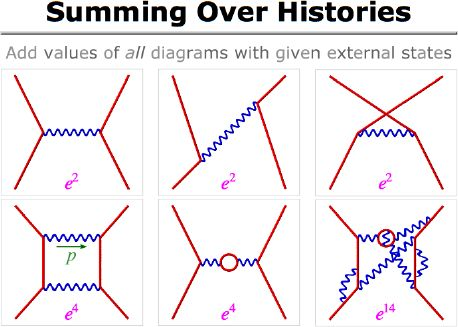# An Introduction to String Theory

Slide 15 of 37<<   [Outline]   >>

So how does all this come together into a physical theory? It turns out that the proper procedure is to construct every possible diagram allowed by the theory (for a given state of input and output particles and how they're moving) and add up the corresponding complex numbers. The result is essentially the "wave function" for that specific input-output state combination, and by squaring that number you can determine the probability that the given input will result in the given output. Doing that is how theorists at particle accelerators earn their keep.

Doing the sums can be a challenge, though, because there are so many possibilities to sum over. First of all, you have to sum over every possible position of the interaction points, summing over all space and time (you even include states where particles seem to move back in time!). You also have to sum over different arrangements of identical particles: in these pictures, for example, an experiment couldn't tell which of the electrons came out which side.

And to make things really complicated, you have to sum over every possible combination of interactions in between: multiple photon exchanges, photons "decaying" into a pair of electrons that then annihilate back into a photon, and much more complicated things. In more complicated sums like this, the sums even have to include every possible way that momentum can be divided between the interacting particles (so, for example, the diagram in the lower left must be summed over every possible momentum of the top photon, since the bottom photon could carry the proper momentum to cancel that out).

The number of diagrams clearly gets bigger and bigger the more interactions you consider, and in general the sum of all those diagrams' values will not converge. A theory like QED works only because the interaction strength e is a small number (in our "c=1, hbar=1" units, e2 is roughly 1/137). Every time you add another pair of interaction vertices, the complex number associated with the diagram is multiplied by that small number, so that horrendous diagram in the lower right hardly makes a difference at all. That is why the sum is able to converge, and it succeeds fantastically well: QED is the best verified theory in physics.

Up to my research page.
Up to my professional page.
My personal site is also available.

Any questions or comments? Write to me: jensens@alma.edu
Copyright © 2004 by Steuard Jensen.